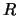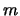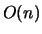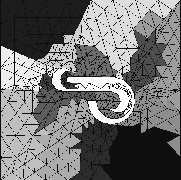Next: Greedy algorithm Up: Graph Theory Based Algorithms Previous: Graph Theory Based Algorithms

### Graph bisection

The recursive graph bisection (RGB) algorithm  attempts to remedy this. Assume that the graph is connected. The RGB algorithm first finds the two vertices that are the furthest apart (their distance is called the diameter of the graph). Then, starting from one of them (the root), the half of the vertices that are closer to the root form one subdomain, the rest form the other. This process is then recursively executed on each of the subdomains.

To find the two vertices that are the furthest apart, a heuristic procedure can be employed. Starting from any vertex as the potential root, label its neighbors with 1, and the neighbors' neighbors as 2, and so on. The last labeled vertex has a label ofwhich is its distance from. Assign this vertex asand repeat the process. When the distancedoes not increase any more,is taken as the root andthe diameter of the graph. In practice this procedure converges quickly in a few iterations.

The graph bisection algorithm has a complexity of. Figure 4 shows the result of applying the RGB algorithm to the dual graph of the mesh of 788 elements, resulting in 142 shared edges, the same as RCB by coincidence.Next: Greedy algorithm Up: Graph Theory Based Algorithms Previous: Graph Theory Based Algorithms

2000-03-21